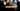# Leetcode | Solution of Subtract the Product and Sum of Digits of an Integer in JavaScript

April 3rd, 2020
|

In this post, we will solve Subtract the Product and Sum of Digits of an Integer from leetcode and compute it's time and space complexities. Let's begin.

# Problem Statement

The question can be found at leetcode Subtract the Product and Sum of Digits of an Integer problem

The problem states that we are given an integer and we need to return the difference between the product and sum of its digits

# Solution

Here's the approach that we'll take

• We'll declare two variables `product` and `sum` and initialize it with `1,0`
• Next, we will loop over all the digits of the number and find their product and sum iteratively
• At last, we will return their difference

We have discussed the approach, I urge you to go ahead on leetcode and give it another try.If you are here, it means something went wrong in implementation or you are just too lazy. In any case, let's see a simple implementation of the above logic.
``````var subtractProductAndSum = function (n) {
let product = 1, sum = 0;

while (n > 0) {
const digit = n % 10;
n = parseInt(n / 10);

product = product * digit;
sum += digit
}

return product - sum
};``````

The only thing to note in the solution is how we find digits of a number. We iterate until the number is greater than zero. Finding modulus of the number by `10` will give us the last digit of the number. Similarly, dividing by `10` would give us the next number. For example - if the number is `123`, modulus `10` would be `3` and divided by `10` would be `12`.

Finally, the number would converge to `0`. At last, we return the difference of the product and sum.

Here are the stats on submission

``````Status: Accepted
Runtime: 52ms
Memory: 34MB``````

## Time and space complexity

### Time complexity

We are looping over the digits of the integer, so time complexity would depend on the number of digits in the number O(len n) or simply O(n)

### Space complexity

We are not using any extra space except for a couple of variables. So space complexity is constant, O(1).

# Summary

So, we solved the Subtract the Product and Sum of Digits of an Integer problem by using a simple while loop and calculated the time and space complexities.

I hope you enjoyed solving this question. This is it for this one, complete source code for this post can be found on my Github Repo. Will see you in the next one.